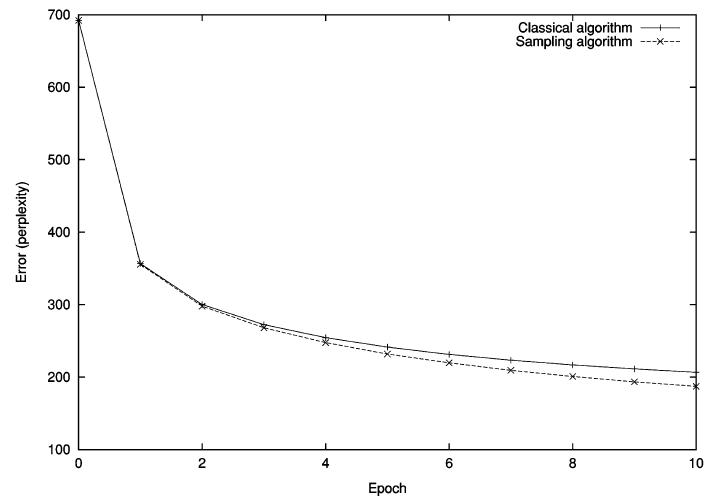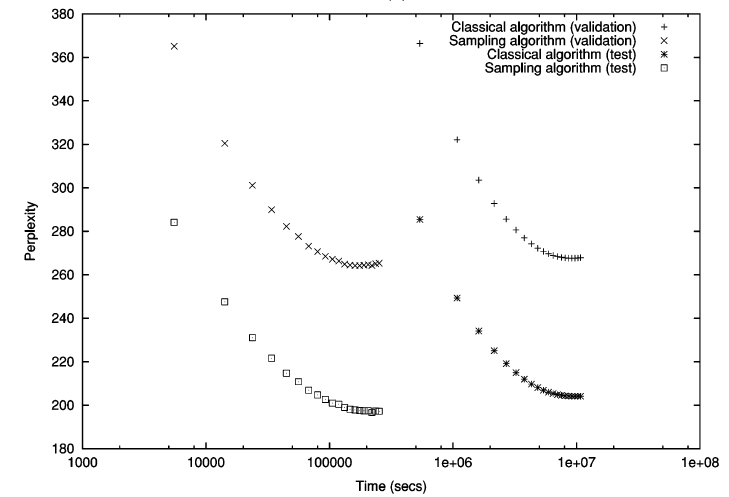# Review — Adaptive Importance Sampling to Accelerate Training of a Neural Probabilistic Language Model

## Feedforward Neural Network for Word Prediction

In this story, Adaptive Importance Sampling to Accelerate Training of a Neural Probabilistic Language Model, by Université de Montréal, is reviewed. This is a paper by Prof. Yoshua Bengio. In this paper:

• A feedforward neural network is trained to approximate probabilities over sequences of words.
• Adaptive importance sampling is designed to accelerate the training.

This is a paper in 2007 TNN with over 200 citations, where TNN has become TNNLS in 2011, and TNNLS has high impact factor of 10.451. (Sik-Ho Tsang @ Medium) Though this paper mainly targets for predicting the next word, word prediction is the foundation to build a language model.

# Outline

1. Neural Language Model Architecture
2. Adaptive Importance Sampling
3. Experimental Results

# 1. Neural Language Model Architecture

• Basically, the network we may think is simple if we compare with the current SOTA approach. Yet, it is amazing at that moment.
• For the existing words wt-1 to wt-n+1, they are transformed to zi using the shared weight C.
• For the next word that needs to predict, a separate D is used to transform it to z0.
• Then, a hidden layer of W (weights) with d (bias) is used with tanh activation to transform z to a:
• Finally, the output is a scalar energy function:
• where bwt is bias and Vwt is the weight from hidden layer to output layer. To obtain the probability:
• where:
• i.e. the softmax operation.

# 2. Adaptive Importance Sampling

## 2.1. Classical Monte Carlo

• At that moment, conventionally, classical Monte Carlo was used to estimate the gradient of the log-likelihood:

## 2.2. Biased Importance Sampling

• In this paper, Biased Importance Sampling is proposed:
• where a multiplicative constant w is used.
• Thus, the gradient updated is scaled.
• which is similar to nowadays weight update procedure.

## 2.3. Effective Sample Size (ESS)

• That is similar to the minibatch size nowadays but ESS is adaptive to w:

# 3. Experimental Results

• Brown corpus dataset is used.
• The Brown corpus consists of 1,181,041 words from various American English documents.
• The corpus was divided in train (800,000 words), validation (200,000 words), and test (the remaining 180,000 words) sets.
• The vocabulary was truncated by mapping all “rare” words (words that appear three times or less in the corpus) into a single special word.
• The resulting vocabulary contains 14,847 words.
• A simple interpolated trigram, serving as baseline, achieves a perplexity of 253.8 on the test set.Training error with respect to number of epochsValidation and test errors with respect to CPU time
• The figure shows that the convergence of both networks is similar. The same holds for validation and test errors.
• The network trained by sampling converges to an even lower perplexity than the ordinary one (trained with the exact gradient).

After 9 epochs (26h), its perplexity over the test set is equivalent to that of the one trained with exact gradient at its overfitting point (18 epochs, 113 days).

• Surprisingly enough, if letting the sampling-trained model converge, it starts to overfit at epoch 18 — as for classical training — but with a lower test perplexity of 196.6, a 3.8% improvement.
• Total improvement in test perplexity with respect to the trigram baseline is 29%.
• The required number of samples with the non-adaptive unigram was growing exponentially.

## Reference

[2007 TNN] [Bengio TNN’07]
Adaptive Importance Sampling to Accelerate Training of a Neural Probabilistic Language Model

## Natural Language Processing (NLP)

Language Model: 2007 [Bengio TNN’07]
Machine Translation: 2014
[Seq2Seq] [RNN Encoder-Decoder] 2015 [Attention Decoder/RNNSearch]
Image Captioning: 2015 [m-RNN] [R-CNN+BRNN] [Show and Tell/NIC]

--

--